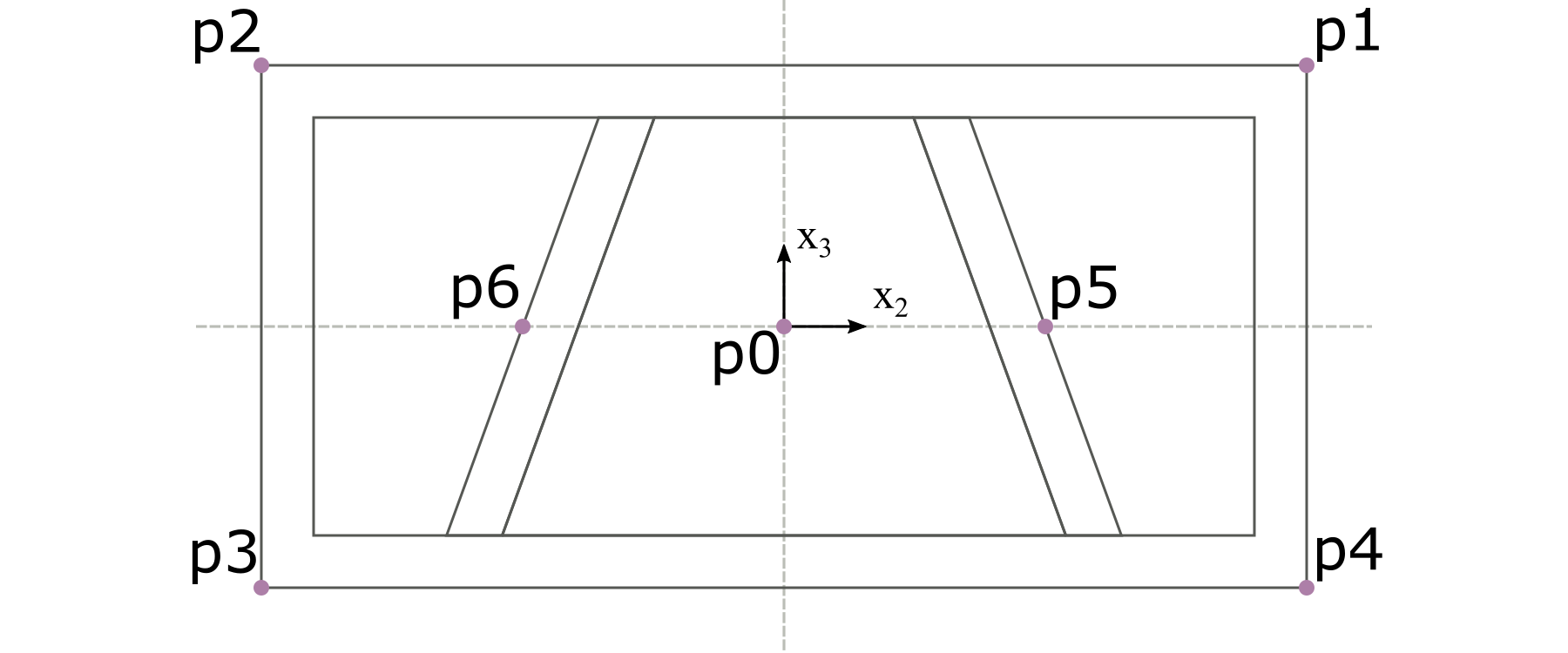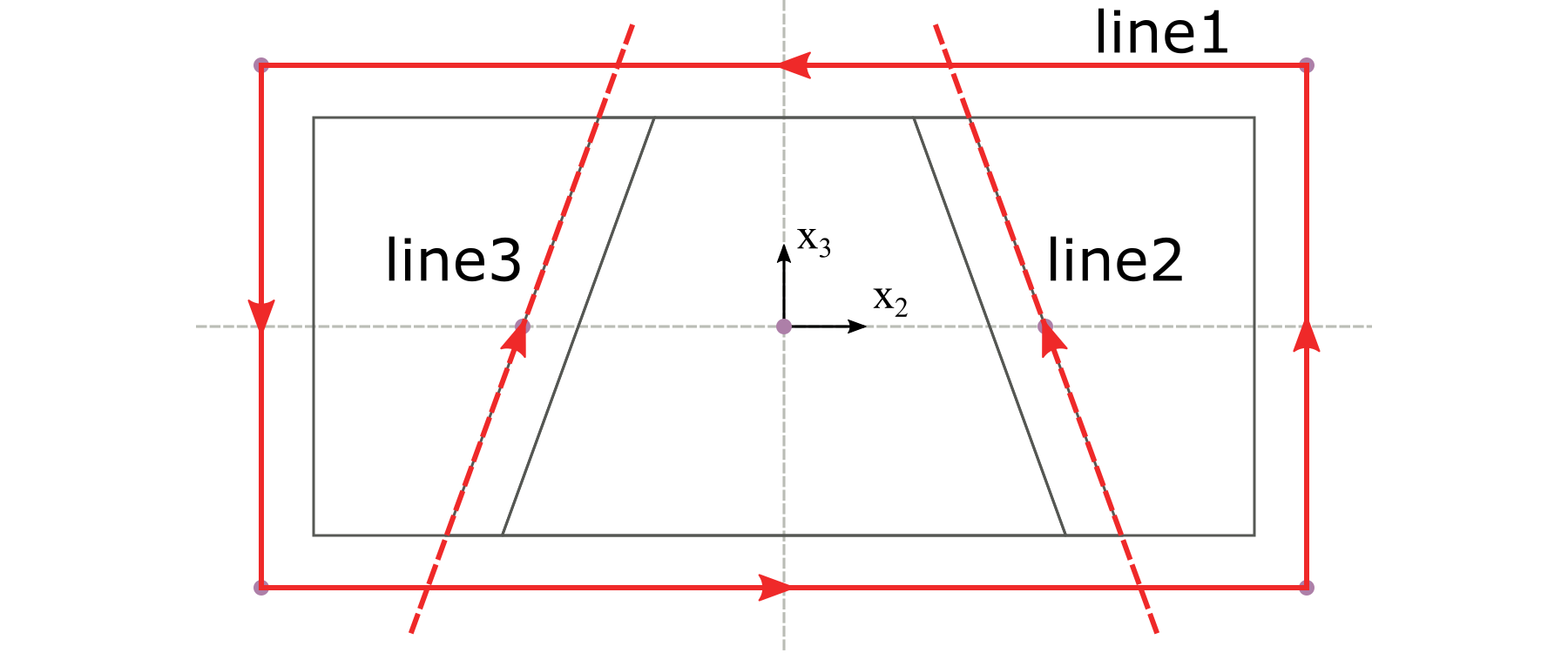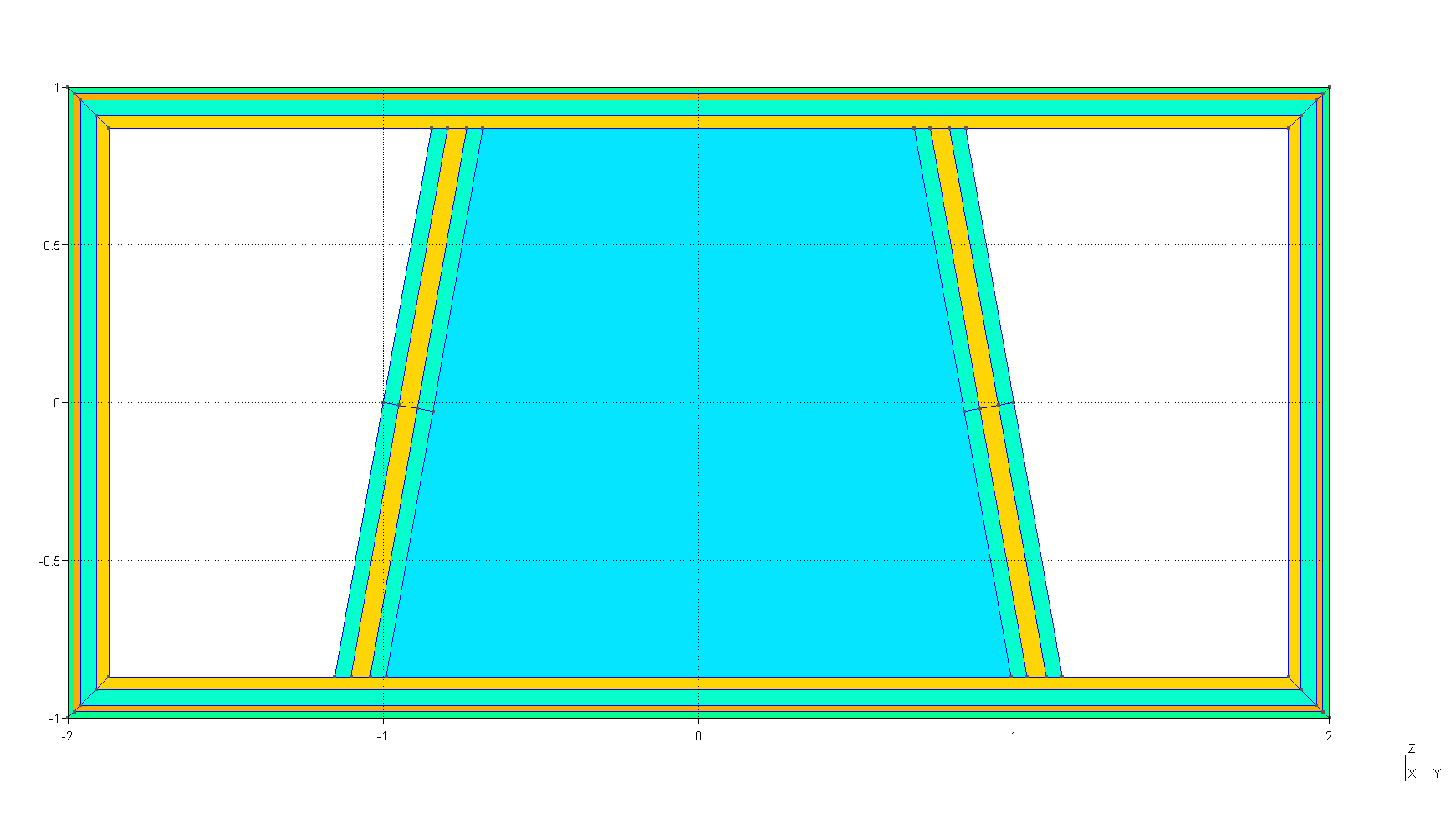# Tutorial#

This tutorial provides a walkthrough for how to prepare input files for PreVABS given a cross section design.

## The design of a cross section#

The box-beam cross section shown in Fig. 23 will be used in this tutorial. All four walls and two webs are made from composite laminates. The two webs are symmetric about a middle vertical line. They are inclined such that the upper portion of both webs are closer to each other than the lower portion. The central space enclosed by the top and bottom walls and two webs is filled with an isotropic material.

The overall shape is described using four parameters, with the following values:

• The width $$w = 4$$ m;

• The height $$h = 2$$ m;

• The distance $$d = 1$$ m;

• The angle $$a = 100^\circ$$.

The materials used in this cross section are listed in Table 34 and Table 35. The layups used in this cross section are listed in Table 36.

Table 34 Isotropic material properties#

Name

Density

$$E$$

$$\nu$$

$$\mathrm{kg/m^3}$$

$$10^3\ \mathrm{Pa}$$

m0

1.00

25.00

0.30

Table 35 Orthotropic material properties#

Name

Density

$$E_{1}$$

$$E_{2}$$

$$E_{3}$$

$$G_{12}$$

$$G_{13}$$

$$G_{23}$$

$$\nu_{12}$$

$$\nu_{13}$$

$$\nu_{23}$$

$$10^3\ \mathrm{kg/m^3}$$

$$\mathrm{GPa}$$

$$\mathrm{GPa}$$

$$\mathrm{GPa}$$

$$\mathrm{GPa}$$

$$\mathrm{GPa}$$

$$\mathrm{GPa}$$

m1

1.86

37.00

9.00

9.00

4.00

4.00

4.00

0.28

0.28

0.28

m2

1.83

10.30

10.30

10.30

8.00

8.00

8.00

0.30

0.30

0.30

Table 36 Layups#

Component

Name

Material

Ply thickness

Orientation

Number of plies

m

degree

Walls

layup_1

m1

0.02

45

1

m1

0.02

-45

1

m2

0.05

0

1

m1

0.02

0

2

Webs

layup_2

m2

0.05

0

1

m1

0.02

0

3

m2

0.05

0

1

## Steps of preparing input files#

In PreVABS, a cross section is composed of components, each of which can either be a laminate or a fill. The laminate type component is composed of segments with different layups. For a realistic cross section, there may be several different ways to decompose it, which may need different information in the input files to build the model.

Hence, the first step is to decide a way of decomposition of the cross section.

For this example, the first step is trivial. The cross section can be decomposed into three components: the walls, the webs, and the fill.

At the same time, the order of creating components should also be decided. This is done by figuring out the dependence relationships between components. For this example, the exact shape of each web depends on the shape of the walls, and the shape of the fill depends on both the webs and the walls. Then the order of creation should be: first creating the walls, then the webs, and at last the fill, as shown in Fig. 24.

The next step is to prepare various input files based on all design parameters listed above. In this example, all files are in the XML format. A brief summary of these input files is listed below.

• A baseline file (baselines.xml), storing definitions of geometric elements.

• A material file (MaterialDB.xml), storing definitions of materials and laminae.

• A layup file (layups.xml), storing definitions of layups.

• A main cross section file (box.xml), storing definitions of components and other configurations of modeling and analysis.

For this tutorial, all files can have arbitrary file names and be placed at any working directory, except the material database, which must be named as MaterialDB.xml and placed at the same location as where the PreVABS executable is.

Another option is to use a local file in the working directory with an arbitrary name storing the material properties. The requirement of using this local file is to explicitly provide the material file name in the main input file. Please check Section: Other input settings.

### Prepare geometric elements#

As shown in Fig. 25, seven points are used to define the shape of the cross section. Points p1 to p4 define the walls, p5 and p6 define the webs, and p0 indicates the space which should be filled with some material. The origin of the coordinate system is placed at the centroid of the rectangular. Based on the design parameters $$w$$, $$h$$ and $$d$$, coordinates of all points are found and listed in Table 37.Figure 25 Key points defining the shape of the cross section.#
Table 37 Key points#

Name

Coordinate

p0

(0, 0)

p1

(2, 1)

p2

(-2, 1)

p3

(-2, -1)

p4

(2, -1)

p5

(1, 0)

p6

(-1, 0)

Base lines are created based on key points defined above. As shown in Fig. 26, three lines are created. Line 1 is defined by connecting the four points (p1 -> p2 -> p3 -> p4 -> p1). Line 2 and 3 are defined by a single point with an incline angle. For line 2, it is the point p5 with an angle of 100 degrees. For line 3, it is the point p6 with an angle of 80 degrees.

The direction of each base line is important. It is related with how the laminate is created for each segment, and how the local coordinate system is defined for each element.

The completed input file for geometry is shown in Listing 29.Figure 26 Base lines defining the shape of the cross section.#
Listing 29 Input file for geometric elements (baseline.xml).#
 1<baselines>
2  <basepoints>
3    <point name="p0">0  0</point>
4    <point name="p1">2  1</point>
5    <point name="p2">-2  1</point>
6    <point name="p3">-2  -1</point>
7    <point name="p4">2  -1</point>
8    <point name="p5">1  0</point>
9    <point name="p6">-1  0</point>
10  </basepoints>
11  <baseline name="line1" type="straight">
12    <points>p1,p2,p3,p4,p1</points>
13  </baseline>
14  <baseline name="line2" type="straight">
15    <point>p5</point>
16    <angle>100</angle>
17  </baseline>
18  <baseline name="line3" type="straight">
19    <point>p6</point>
20    <angle>80</angle>
21  </baseline>
22</baselines>


### Prepare materials and layups#

Material data are stored in the material database. As stated above, this file must be named as MaterialDB.xml and placed at the directory where the PreVABS executable is. The format of this file is shown in Listing 30. Arrangement of data under the <elastic> element are different for different material types, which can be isotropic, orthotropic, or anisotropic.

Another part of the file is the lamina data. A lamina is a unique combination of material and ply thickness. For this example, according to the layup table (:Table 36) given above, there are two laminae. One has material m1 and thickness 0.02, and another one has material m2 and thickness 0.05. It is the lamina, instead of the material, that will be used to define each layer of the layup.

Listing 30 Input file for materials (MaterialDB.xml).#
 1<materials>
2  <material name="m0" type="isotropic">
3    <density>1.0</density>
4    <elastic>
5      <e>25.0e3</e>
6      <nu>0.3</nu>
7    </elastic>
8  </material>
9  <!-- =========================================================== -->
10  <material name="m1" type="orthotropic">
11    <density>1.86E+03</density>
12    <elastic>
13      <e1>3.70E+10</e1>
14      <e2>9.00E+09</e2>
15      <e3>9.00E+09</e3>
16      <g12>4.00E+09</g12>
17      <g13>4.00E+09</g13>
18      <g23>4.00E+09</g23>
19      <nu12>0.28</nu12>
20      <nu13>0.28</nu13>
21      <nu23>0.28</nu23>
22    </elastic>
23  </material>
24  <lamina name="la_m1_002">
25    <material>m1</material>
26    <thickness>0.02</thickness>
27  </lamina>
28  <!-- =========================================================== -->
29  <material name="m2" type="orthotropic">
30    <density>1.83E+03</density>
31    <elastic>
32      <e1>1.03E+10</e1>
33      <e2>1.03E+10</e2>
34      <e3>1.03E+10</e3>
35      <g12>8.00E+09</g12>
36      <g13>8.00E+09</g13>
37      <g23>8.00E+09</g23>
38      <nu12>0.30</nu12>
39      <nu13>0.30</nu13>
40      <nu23>0.30</nu23>
41    </elastic>
42  </material>
43  <lamina name="la_m2_005">
44    <material>m2</material>
45    <thickness>0.05</thickness>
46  </lamina>
47</materials>


Layup information is stored in a separate file. Based on the layup table, the input file can be prepared with the format shown in Listing 31. The order of the layers defines the laying sequence from the base line. The number in the <layer> element stands for the orientation. If there is a colon, then the number behind it stands for the number of plies in this layer. If there is no number at all, then by default this layer has only one ply with orientation of 0 degree.

Listing 31 Input file for layups (layups.xml).#
 1<layups>
2  <layup name="layup1">
3    <layer lamina="la_m1_002">45</layer>
4    <layer lamina="la_m1_002">-45</layer>
5    <layer lamina="la_m2_005">0</layer>
6    <layer lamina="la_m1_002">0:2</layer>
7  </layup>
8  <layup name="layup2">
9    <layer lamina="la_m2_005"></layer>
10    <layer lamina="la_m1_002">0:3</layer>
11    <layer lamina="la_m2_005"></layer>
12  </layup>
13</layups>


### Create components#

There are two types of components in PreVABS, laminate and fill. For this example, based on the decomposition we did at the begining of this section, there are three laminate-type components and one fill-type component. Besides, the sequence of creating components is important as stated at the beginning of this section. This is defined by declaring which components are needed before creating the current one.

Each laminate-type component is composed of one or more segments. Each segment is a unique combination of a base line and a layup. The layup can be created on either side of the base line. This is controlled by an attribute direction, which can be either left or right. This can be understood as the left- or right-hand side when walking along the base line, as shown in Fig. 27.

As for the ordering, the walls should be completed first, since its inner boundary is needed to trim the base lines of both webs. Hence, each web component depends on the walls component, as shown in Listing 32.

Listing 32 Input elements for the laminate-type components#
 1<component name="walls">
2  <segment>
3    <baseline>line1</baseline>
4    <layup>layup1</layup>
5  </segment>
6</component>
7<component name="web1" depend="walls">
8  <segment>
9    <baseline>line2</baseline>
10    <layup>layup2</layup>
11  </segment>
12</component>
13<component name="web2" depend="walls">
14  <segment>
15    <baseline>line3</baseline>
16    <layup direction="right">layup2</layup>
17  </segment>
18</component>


The fill-type component is defined by a point and a material. Here, the point p0 is used to indicate that the material should be filled in the space in the middle enclosed by the walls and both webs. At the same time, the dependent components are also decided, as shown in Listing 33.

Listing 33 Input elements for the fill-type component#
1<component name="fill" type="fill" depend="walls,web1,web2">
2  <location>p0</location>
3  <material>m0</material>
4</component>


### Set other configurations and complete the main input file#

Besides the definitions of components, the main input file of the cross section contains several other required or optional settings.

The first part is the <include> settings, which is required. This contains names of the base lines and layups files.

The second part is the <analysis> settings, which is optional. This contains configurations used by VABS for the cross-sectional analysis. For this example, the <model> setting is set to 1, which means that the Timoshenko beam model will be used and the 6x6 stiffness matrix will be calculated.

The last part is the <general> settings, which is optional. This part contains global configurations for the shape and meshing of the cross section. Here, the global mesh size is set to 0.02.

The completed main input file for the cross section is shown in Listing 34.

Listing 34 Input file for the cross section#
 1<cross_section name="box">
2  <include>
3    <baseline>baselines</baseline>
4    <layup>layups</layup>
5  </include>
6  <analysis>
7    <model>1</model>
8  </analysis>
9  <general>
10    <mesh_size>0.02</mesh_size>
11  </general>
12  <component name="walls">
13    <segment>
14      <baseline>line1</baseline>
15      <layup>layup1</layup>
16    </segment>
17  </component>
18  <component name="web1" depend="walls">
19    <segment>
20      <baseline>line2</baseline>
21      <layup>layup2</layup>
22    </segment>
23  </component>
24  <component name="web2" depend="walls">
25    <segment>
26      <baseline>line3</baseline>
27      <layup direction="right">layup2</layup>
28    </segment>
29  </component>
30  <component name="fill" type="fill" depend="walls,web1,web2">
31    <location>p0</location>
32    <material>m0</material>
33  </component>
34</cross_section>


## Execution and results#

Once all input files are prepared, the cross section can be created and homogenized using the following command:

prevabs -i box.xml -h -v -e


If everything works successfully, Gmsh will be called and the cross section will be plotted as shown in Fig. 29 (the display of element edges is turned off in the figure for clarity), and VABS homogenization analysis will be carried out and effective beam properties can be found in the file box.sg.K. The effective Timoshenko stiffness matrix is listed in Table 38.Figure 29 The cross section created by PreVABS and plotted by Gmsh.#
 3.991e+10 -166200 -40.37 4.204e+07 -4358 28.67 -166200 6.743e+09 39450 -2.918e+08 -1.577e+07 -4150 -40.37 39450 6.165e+09 8220 -2897 -8.7e+06 4.204e+07 -2.918e+08 8220 1.972e+10 272100 330.3 -4358 -1.577e+07 -2897 272100 2.173e+10 -30250 28.67 -4150 -8.7e+06 330.3 -30250 6.728e+10